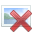# Coupled RC circuits with AC current source

• Engineering
no_alone

## Homework Statement

Hi, I already asked a question close to this, but now I have different conditions.
This is the circuit$C_1 = C_2 \\ R_1= R_2$

The current is an AC , and I would like to know the voltage at R_1 and at R_2
I made some progress but I do not really know hot to continue.

## The Attempt at a Solution

VR1 = VC1
VR1 + VRc - VC2 = 0
VR2 = VC2
IR1 + IC1 + VRC = Iinj
IRc = IR2 + VC2
$$V_1 = V_{C1} = V_{R1} \\ V_2 = V_{R2} = V_{C2}$$

$$\frac{V_1}{R_1} + \frac{dV_1}{dt}*C_1 + \frac{V_{Rc}}{R_c} = I_{inj} \\ \frac{V_{Rc}}{R_c} = \frac{V_2}{R_2} + C_2*\frac{dV_2}{dt}\\$$

$$\frac{dV_1}{dt}*C_1 = I_{\omega} -\frac{V_1-V_2}{R_c} - \frac{V_1}{R_1} \\ \frac{dV_2}{dt}*C_2 = -\frac{V_2}{R_2} +\frac{V_1-V_2}{R_c} \\ --> C_1 == C_2 , R_1 == R_2 \\ \frac{dV_1}{dt} = \frac{I_{\omega}}{C_1} -\frac{V_1-V_2}{R_c*C_1} - \frac{V_1}{R_1*C_1} \\ \frac{dV_2}{dt} = -\frac{V_2}{R_1*C_1} +\frac{V_1-V_2}{R_c*C_1} \\$$

## Answers and Replies

Mentor
With AC sources you can obtain steady-state solutions for such circuits using impedances for the components and phasor values for the sources. No need to write or solve differential equations, the usual DC circuit methods and techniques of analysis will work fine.

Homework Helper
Gold Member

## The Attempt at a Solution

IR1 + IC1 + VRC = Iinj
IRc = IR2 + VC2
You can't have voltage and current terms in the same equation. Straightening this out is your first task.

It is perectly OK to use differential equations as you have done, especially if you never had phasors..